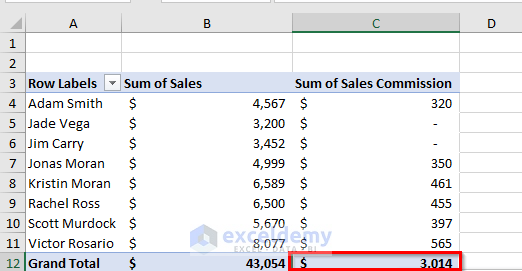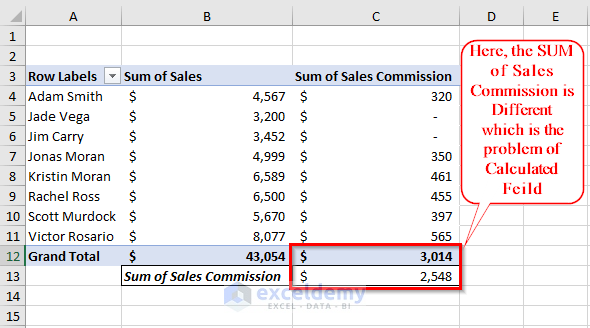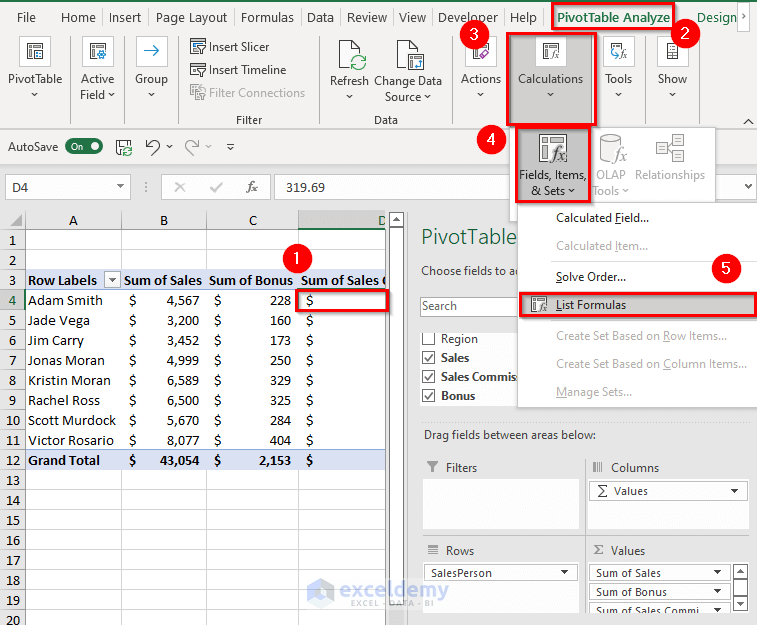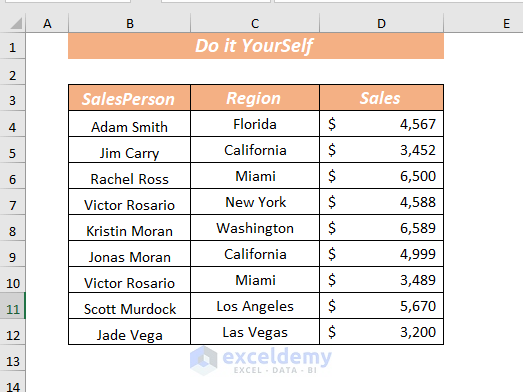# How to Use Calculated Field in Excel Pivot Table (8 Ways)

Get FREE Advanced Excel Exercises with Solutions!

In Excel you can create a Pivot Table from any dataset, Pivot Table is useful when you need a new data point that can be obtained by using existing data points in the Pivot Table. Here you won’t need to go back and add it to the source data. Instead, by using a Calculated Field you can do this. A calculated field is created by using formulas in the Pivot Table. In this article, I’m going to explain how you can use the calculated field in Pivot Table.

To make the explanation understandable, I’m going to use a sample dataset that represents the sales information of a particular salesperson. The dataset has 3 columns; these are SalesPerson, Region, and Sales.## 8 Ways to Use Calculated Field in Pivot Table

### 1. Create A Pivot Table

Before diving into the adding of Calculated Field let me show you the process of creating a Pivot Table.

To create a Pivot Table, I’m going to use the dataset given below.To start with, select the cell range from where you want to create a Pivot Table.

➤ I selected the cell range B3:D12.

Now, open the Insert tab >> from PivotTable >> select From Table/RangeA dialog box will pop up. From there choose the place to place your PivotTable.

⏩ I selected New Worksheet.

Next, click OK.➤Then, a new sheet of PivotTable will open.

Now, choose the field from PivotTable Fields that you want to display in the PivotTable layout.

⏩ I selected the SalesPerson in Rows and Sales in Values.Hence, you will get the selected field in the PivotTable layout.### 2. Inserting Simple Calculated Field in Pivot Table

As my PivotTable is ready now, I’m going to show you the process of adding a simple Calculated Field.

Here, I want to add a field named Bonus depending on Sales information. The bonus amount will be 5% of the sales individual SalesPerson achieved.

To begin with, select any cell from the Pivot Table.

➤ I selected cell B4.

Now, open the PivotTable Analyze tab >> go to Calculations >> from Fields, Items, & Sets >> select Calculated FieldA dialog box will pop up. From there insert Name and Formula.

⏩ I used Bonus in Name.

Type the following formula in Formula.

`=Sales*0.05`

Here, Sales values are multiplied by 5%.As the Formula is added then click OK.Therefore, you will get the Calculated Field name Bonus in the PivotTable.Here all Bonuses of individual SalesPerson are calculated automatically just by creating a Calculated Field.

### 3. Adding Complex Calculated Field in Pivot Table

If you want, you also can add a complex Calculated Field. Where you can use different functions to calculate any field depending on the existing values of the Pivot Table.

To demonstrate the procedure, I’m going to use an IF function to calculate the Commission based on Sales. If any particular SalesPerson’s Sales amount is greater than (>) \$5000 then he/she will get 8% of the commission.

To begin with, select any cell from the Pivot Table.

➤ I selected cell C4.

Now, open the PivotTable Analyze tab >> go to Calculations >> from Fields, Items, & Sets >> select Calculated FieldA dialog box will pop up. From there insert Name and Formula.

⏩ I used Sales Commission in Name.

Type the following formula in Formula.

`=IF(Sales>5000,Sales*8%, 0)`

Here, in the IF function, I used Sales>5000 as logical_test, Sales*8% as value_if_true and used 0 as value_if_false. If the condition is met, then it will return the commission of 8% of Sales otherwise 0.As the Formula is added then click OK.As a result, you will get the Calculated Field name Sales Commission in the PivotTable.Here, all Sales Commissions of individual SalesPerson are calculated automatically just by creating a Calculated Field.

### 4. Modify an Existing Calculated Field

It may happen that you may need to modify or change the Calculated Field. In those cases, you won’t need to worry because there is an option named Modify in the Calculated Field.

Here, I want to modify the field Sales Commission. Instead of 8%, I want to provide a 7% commission where sales value is greater than \$4500.➤ I selected cell C4.

Now, open the PivotTable Analyze tab >> go to Calculations >> from Fields, Items, & Sets >> select Calculated FieldA dialog box will pop up. Select Sales Commission from Name to see the existing Formula.From the dialog box, you can modify your existing Formula.

⏩ Type the following formula in Formula.

`=IF(Sales>4500,Sales*7%, 0)`

Here, in the IF function, I used Sales>4500 as logical_test, Sales*7% as value_if_true and used 0 as value_if_false. If the condition is met, then it will return the commission of 7% of Sales otherwise 0.As Formula is modified so click OK.Finally, you will get the modified values in the Calculated Field name Sales Commission in the PivotTable.Here all Sales Commissions of individual SalesPerson are modified automatically based on the given Formula.

### 5. Drawback of Calculated Field in Pivot Table

Though Calculated Field is easier to use and calculates the fields’ values automatically, yet it has an issue while calculating the SUM of the Calculated Amounts.

Carefully look at the Sum of Sales Commission which shows 3014 dollars. Let’s calculate the SUM manually using the SUM function.

###To calculate the SUM of Sales Commission, select any cell to place your resultant value.

➤ I selected cell C13.

In cell C13 type the following formula.

`=SUM(C4:C11)`Here, the SUM function will add all the available values of the selected range C4:C11.

Now, press the ENTER key to get the SUM.

Hence, you will get the SUM of the Sales Commission.

###The Grand Total I’ve gotten from the Calculated Field is 3014 and the Grand Total I’ve gotten from the SUM function is 2548.

This means the Grand Total of the Calculated Field is incorrect for the Sales Commission field. Here, the Grand Total is not the SUM rather it’s the 7% of the Grand Total of Sales. Because the Calculated Field uses the same calculation in the SubTotal and Grand Total rows, instead of showing a SUM.

#### 5.1. Ways to Avoid Calculation Problem of Calculated Field

Now, I’m going to provide a couple of ways to avoid such types of misleading calculations of Calculated Field.

You can use the Filter option to avoid the calculation problem. By using the Filter option I’ll hide the values that are not greater than 4500.

To apply the Filter, select the Row Labels and expand the Filter options.

From Value Filters >> select Greater Than.In Show items for which provide the condition you already used in your Calculated Field.

➤ I selected “Sum of Sales” “is greater than” “4500”.

Then, click OK.Hence, you will get the Grand Total of the values which met the condition applied on the Calculated Field.

The Grand Total of Sales Commission is 2548.If you want, you can recheck it for confirmation.

To calculate the SUM of Sales Commission, select any cell to place your resultant value.

➤ I selected cell C13.

In cell C13 type the following formula.

`=SUM(C4:C9)`Here, the SUM function will add all the available values of the selected range C4:C9.

Now, press the ENTER key to get the SUM.

Hence, you will get the SUM of the Sales Commission.Here both Grand Total and SUM are equal.

In case you don’t require the Grand Total then you can remove the Grand Total from the sheet.

Select Grand Total then right click on the mouse.

A context menu will appear from there select Remove Grand Total.Here, the Grand Total is removed from the sheet.If you want, you can calculate the Grand Total outside the PivotTable just as I did to get the SUM of the Sales Commission.

### 6. Get the List of All the Calculated Field Formulas

The Pivot Table has a built-in command named List Formulas which helps us to get the Formulas that we used in PivotTable.

To get the list of formulas,

Open the PivotTable Analyze tab >> go to Calculations >> from Fields, Items, & Sets >> select List Formulas

###All the used formulas will appear in a new sheet.### 7. Temporarily Remove Pivot Table Calculated Field

In any case, you may wish to or need to remove a Calculated Field temporarily.

From the dataset given below, I want to remove the Sum of Bonus Calculated Field temporarily.To begin with, select any cell from the Calculated Field that you want to remove.

➤ I selected cell C3.

Now, right click on the mouse >> from the Context Menu >> select Remove “Sum of Bonus”Here, the Calculated Field Sum of Bonus is removed.Though the Sum of Bonus field is removed from the PivotTable layout but it is still available in PivotTable Fields. You can use it again if you want.You also can uncheck the Calculated Field from the PivotTable Fields to remove the Calculated Field temporarily.

### 8. Permanently Remove A Calculated Field from Pivot Table

You also can remove a Calculated Field permanently if you are sure that you don’t need the field anymore.

To begin with, select any cell from the Calculated Field that you want to remove permanently.

➤ I selected cell C4.

Now, open the PivotTable Analyze tab >> go to Calculations >> from Fields, Items, & Sets >> select Calculated Field.A dialog box will pop up. Select Bonus from Name to see the existing Formula.

Now, click on Delete.Next, click OK.Therefore, the Sum of Bonus field is removed permanently from the PivotTable layout as well as from the PivotTable Fields.## Things to Remember

🔺 Remember to remove 0 from the Formula before inserting a formula for calculation. You can use Formulas that don’t require cell references.

🔺 While using Calculated Field you will need to check the Sub Totals and Grand Totals.

## Practice Section

I’ve provided a practice sheet in the workbook to practice these explained examples.

#### Conclusion

In this article, I have explained several ways how you can use calculated field in pivot table. I also explained the drawback as well as the reasons why the calculated field fails to work. Last but not least, if you have any kind of suggestions, ideas, or feedback please feel free to comment down below.

## Related ArticlesShamima Sultana

Hello! Welcome to my Profile. I am Shamima Sultana Rita. Currently, I'm working as a Project Manager at ExcelDemy. I am doing research on Microsoft Excel and here we will be posting articles related to this. My last educational degree was BSc and my program was in Computer Science and Engineering from East West University-Bangladesh. I am a Computer Science graduate with a great interest in research and development. I love to learn new things. Data Analysis is one of my favorite fields as I love to extract patterns based on problems. Here, I'm trying to provide enriched quality content regarding Excel basics to Advanced. Always try to gather knowledge from various sources and try to make innovative solutions.

We will be happy to hear your thoughtsAdvanced Excel Exercises with Solutions PDF